# Program to check Emirp Number in Java

In this tutorial you will learn about the Program to check Emirp Number in Java and its application with practical example.

In this tutorial, we will learn to create a Java Program to check Emirp Numbers in Java using Java programming.

## Prerequisites

Before starting with this tutorial we assume that you are best aware of the following Java programming topics:

• Java Operators.
• Basic Input and Output function in Java.
• Class and Object in Java.
• Basic Java programming.
• If-else statements in Java.
• For loop in Java.

## What is Emirp Number?

A prime number 79 means that divisible by 1 and itself only. On overturn the number, we get a number 97 which is also a prime number. so we can say that number 79 and 97 both are prime numbers.

Example:

“17  = 71” both are Prime number so they are Emirp number

“19  = 91 ” Both are not Prime number so they are notEmirp number.

“79  = 97”  both are prime number so they are Emirp number.

## Program to check Emirp Number in Java

In this our program we will find given number is a Emirp number or not in using java programming. We would first declared and initialized the required variables. Next, we would prompt user to input the a number.Later we  find number is Emirp  number or not let’s have a look at the code.

## Output

Emirp Number.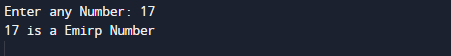Not a Emirp Number.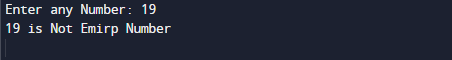In the above program, we have first declared and initialized a set variables required in the program.

• number= it will hold entered number.

After declaring variables in the next statement user will be prompted to enter a value and  which will be assigned to variable ‘number’.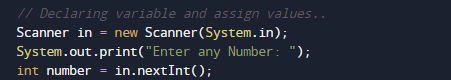## Logic to find Emirp Number

• take a number form user and  initialize the number (number) to a variable
• First of all find given number is number prime or not.• If not, break the execution and exit.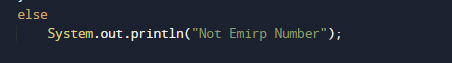• If number is a prime,then find the reverse (reverse ) of the given number (number).as shown in image below.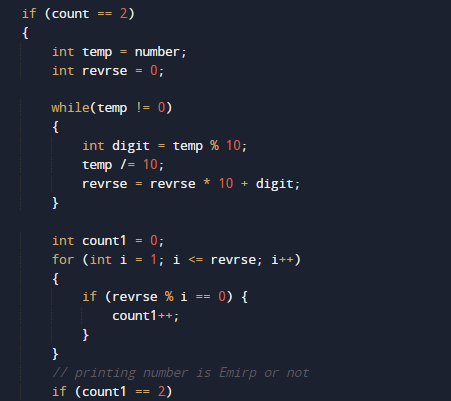• Now reverse  the number (reverse) is prime or not as shown above.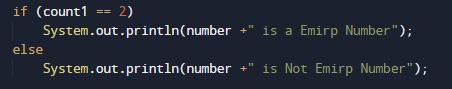• If not, print number (n) is not emirp.

In this tutorial we have learn about the Program to check Emirp Number in Java and its application with practical example. I hope you will like this tutorial.